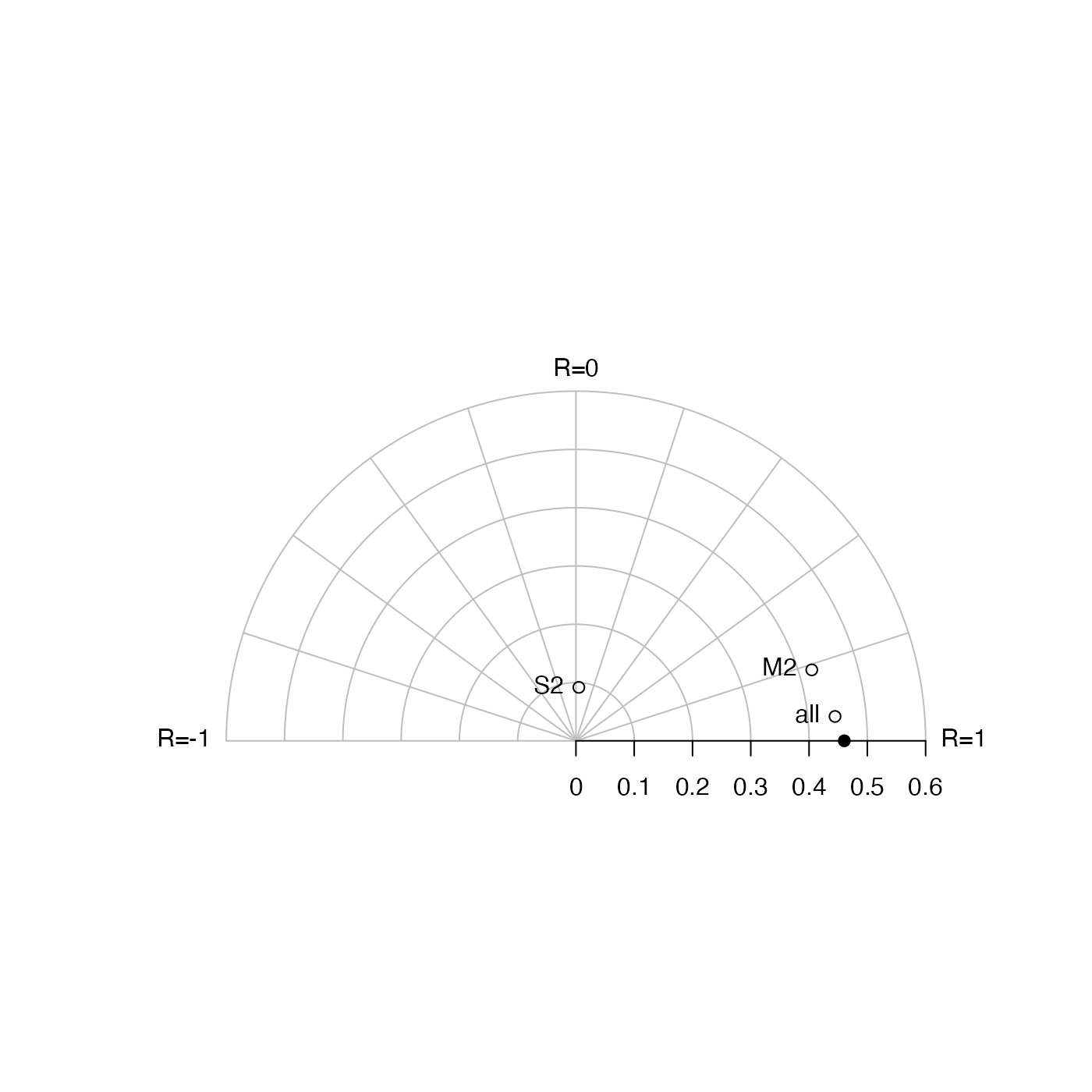Creates a diagram as described by Taylor (2001). The graph is in the form of a semicircle, with radial lines and spokes connecting at a focus point on the flat (lower) edge. The radius of a point on the graph indicates the standard deviation of the corresponding quantity, i.e. x and the columns in y. The angle connecting a point on the graph to the focus provides an indication of correlation coefficient with respect to x. The east'' side of the graph indicates $$R=1$$, while $$R=0$$ is at the "north" edge and $$R=-1$$ is at the "west" side. The x data are indicated with a bullet on the graph, appearing on the lower edge to the right of the focus at a distance indicating the standard deviation of x. The other points on the graph represent the columns of y, coded automatically or with the supplied values of pch and col. The example shows two tidal models of the Halifax sealevel data, computed with tidem() with just the M2 component and the S2 component; the graph indicates that the M2 model is much better than the S2 model.

plotTaylor(x, y, scale, pch, col, labels, pos, ...)

## Arguments

x a vector of reference values of some quantity, e.g. measured over time or space. a matrix whose columns hold values of values to be compared with those in x. (If y is a vector, it is converted first to a one-column matrix). optional scale, interpreted as the maximum value of standard deviation. list of characters to plot, one for each column of y. list of colors for points on the plot, one for each column of y. optional vector of strings to use for labelling the points. optional vector of positions for labelling strings. If not provided, labels will be to the left of the symbols. optional arguments passed by plotTaylor to more child functions.

Taylor, Karl E., 2001. Summarizing multiple aspects of model performance in a single diagram, J. Geophys. Res., 106:D7, 7183--7192.

## Examples

library(oce)
data(sealevel)
x <- sealevel[["elevation"]]
M2 <- predict(tidem(sealevel, constituents="M2"))
S2 <- predict(tidem(sealevel, constituents=c("S2")))
plotTaylor(x, cbind(M2, S2))